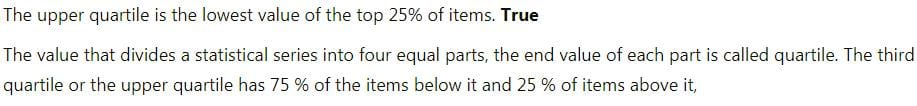# Test: Measures Of Central Tendency

## 10 Questions MCQ Test Economics Class 11 | Test: Measures Of Central Tendency

Description
Attempt Test: Measures Of Central Tendency | 10 questions in 10 minutes | Mock test for Commerce preparation | Free important questions MCQ to study Economics Class 11 for Commerce Exam | Download free PDF with solutions
QUESTION: 1

### Upper quartile is the lowest value of

Solution:QUESTION: 2

### Arithmetic mean is of

Solution:

Arithmetic mean is of two types:

• Simple arithmetic mean
• Weighted mean.
QUESTION: 3

### Deciles refer to the division of series into

Solution:

Deciles, as its name suggests, refers to the division of series into ten equal parts. The value of each part is known as deciles.

QUESTION: 4

The another name of the 'measure of central tendency' is called

Solution:

Measures of central tendency refers to all those methods of statistical analysis which are used to calculate the average of a set of data.

QUESTION: 5

Arrangment of data in the given series is required while computing

Solution:

Median requires arrangement of data in either ascending or descending order before calculating median.

QUESTION: 6

Lower limit of first group and upper limit of last group are undefined in

Solution:

In case of classes like, below 10, 10-20, 20-30, 30-40, 40-50, above 50, computation of arithmetic mean would be impossible unless we assume the unknown limits.

QUESTION: 7

Which of the following is not a measure of central tendency?

Solution:

► Standard deviation is the measure of how spread out the numbers of a data are.

► Mean is the average.

► Median is the middle number, when data is arranged in numerical order.

► Mode is the data item the appears most frequently.

Mean, median and mode are all measures of central tendencies.

QUESTION: 8

The mathematical average is also called

Solution:

► Mean is a mathematical average.

The arithmetic mean or mean is defined as the sum of values of a group of items divided by the number of items. It is denoted by mean.

QUESTION: 9

Calculation of mean is very difficult in case of

Solution:

In case of open end classes, computation of arithmetic mean would be difficult unless we assume the unknown limits.

QUESTION: 10

Value that divides the series into hundred equal parts is called

Solution:

Values that divide the series into hundred equal parts are called percentiles. In percentile, we get 99 dividing positions denoted by P1, P2,……..,P99.Use Code STAYHOME200 and get INR 200 additional OFF Use Coupon Code